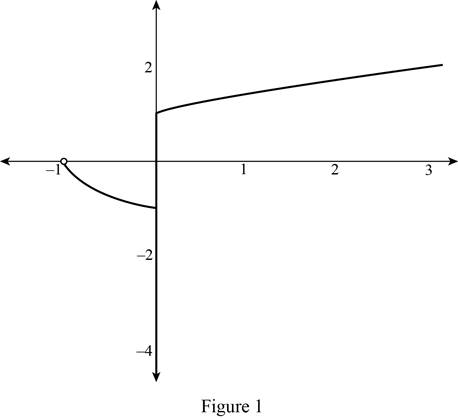# To estimate: The value of lim x → 0 − x 2 + x x 3 + x 2 from the graph of f ( x ) = x 2 + x x 3 + x 2 .### Single Variable Calculus: Concepts...

4th Edition
James Stewart
Publisher: Cengage Learning
ISBN: 9781337687805### Single Variable Calculus: Concepts...

4th Edition
James Stewart
Publisher: Cengage Learning
ISBN: 9781337687805

#### Solutions

Chapter 2.2, Problem 10E

(a)

To determine

## To estimate: The value of limx→0−x2+xx3+x2 from the graph of f(x)=x2+xx3+x2.

Expert Solution

The value of limx0x2+xx3+x2=1.

### Explanation of Solution

Using the graphing calculator, the graph of the function f(x)=x2+xx3+x2 is drawn and shown below in Figure 1.From Figure 1, it is observed that the graph approaches y=1 as x approaches 0 from the left side.

Thus, the value of limx0x2+xx3+x2=1.

(b)

To determine

### To estimate: The value of limx→0+x2+xx3+x2 from the graph of f(x)=x2+xx3+x2.

Expert Solution

The value of limx0+x2+xx3+x2=1.

### Explanation of Solution

From Figure 1, it is observed that the graph approaches y=1 as x approaches 0 from the right side.

Thus, the value of limx0+x2+xx3+x2=1.

(c)

To determine

### To estimate: The value of limx→0x2+xx3+x2 from the graph of f(x)=x2+xx3+x2.

Expert Solution

The value of limx0x2+xx3+x2 does not exist.

### Explanation of Solution

From Figure 1, it is observed that the graph does not approach any value when x equals exactly 0.

Also, notice the left hand limit and the right limit of limx011+e1x obtained from part (a) and part (b) are not the same.

That is limx0x2+xx3+x2=1 and limx0+x2+xx3+x2=1.

Thus, it is concluded that limx0x2+xx3+x2 does not exist.

### Have a homework question?

Subscribe to bartleby learn! Ask subject matter experts 30 homework questions each month. Plus, you’ll have access to millions of step-by-step textbook answers!